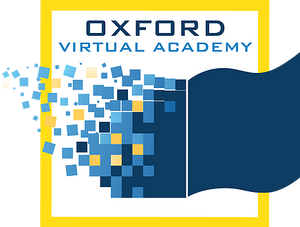# Pre-Algebra

Pre-Algebra, provides the basis for the course content. The student will solve equations and inequalities with positive and negative integers, decimals, and fractions. The student will then use the algebra skills to work with ratios, proportions, and percentages. In the second course, the student will explore basic algebraic principles. Students will also examine and evaluate two-step and multi-step equations and inequalities and then explore and use graphs to solve linear relations and functions. Next, the students will be introduced to basic concepts of geometry including angle relationships, parallel lines, polygons, circles, and transformations. The student will continue to apply his knowledge of geometry and algebra to solve area and volume problems. Then the student will explore nonlinear functions and polynomials. Finally, the student will examine properties of right triangles, data analysis, and probability.

* Prerequisites: Math 6 or Math 7